# D.2 模型拟合程度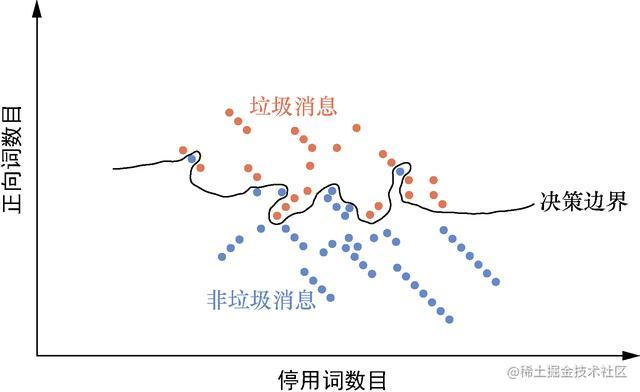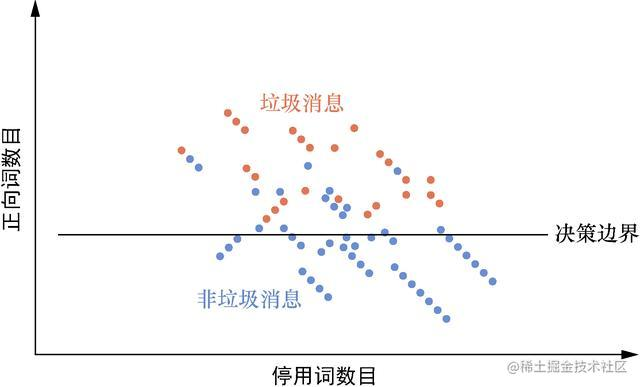# D.4 交叉拟合训练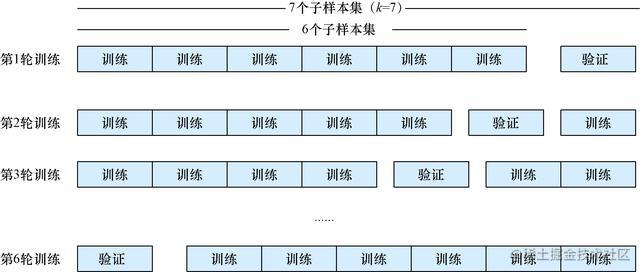• 正则化；
• 随机dropout；
• 批归一化。

# D.5.1 正则化

L1正则化：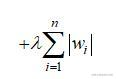L1是所有参数（权重）的绝对值与某个λ（超参数）乘积的和，通常是0到1之间的一个小浮点数。这个和应用于权重的更新——其思想是，较大的权重会产生较大的惩罚，因此鼓励模型使用更多的、均匀的权重……

L2正则化：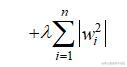# D.5.2 dropout

Keras提供了一种非常简单的实现方法，可以在本书的示例和代码清单D-1中看到。

``````>>> from keras.models import Sequential
>>> from keras.layers import Dropout, LSTM, Flatten, Dense
>>> num_neurons = 20
>>> maxlen = 100
>>> embedding_dims = 300
>>> model = Sequential()
...                input_shape=(maxlen, embedding_dims)))

# D.5.3 批归一化

``````>>> from keras.models import Sequential
>>> from keras.layers import Activation, Dropout, LSTM, Flatten, Dense
>>> from keras.layers.normalization import BatchNormalization
>>> model = Sequential()

# D.6.3 数据增强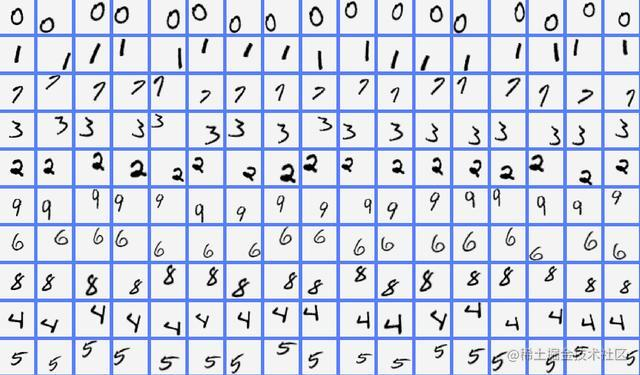# D.7.1 分类的衡量指标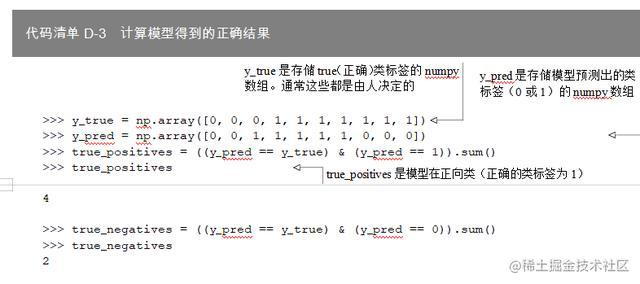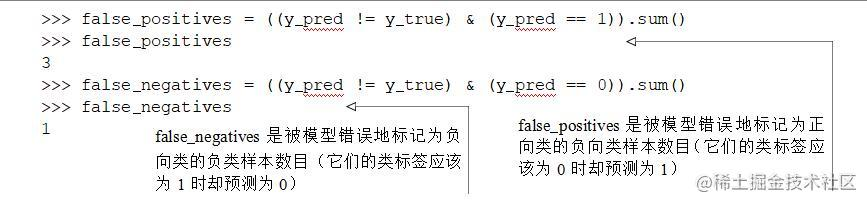``````>>> confusion = [[true_positives, false_positives],
...              [false_negatives, true_negatives]]
>>> confusion
[[4, 3], [1, 2]]
>>> import pandas as pd
>>> confusion = pd.DataFrame(confusion, columns=[1, 0], index=[1, 0])
>>> confusion.index.name = r'pred \ truth'
>>> confusion
1   0
pred \ truth
1             4   1
0             3   2

``````>>> precision = true_positives / (true_positives + false_positives)
>>> precision
0.571...

``````>>> recall = true_positives / (true_positives + false_negatives)
>>> recall
0.8

# D.7.2 回归的衡量指标

``````>>> y_true = np.array([0, 0, 0, 1, 1, 1, 1, 1, 1, 1])
>>> y_pred = np.array([0, 0, 1, 1, 1, 1, 1, 0, 0, 0])
>>> rmse = np.sqrt((y_true - y_pred) ** 2) / len(y_true))
>>> rmse
0.632...

``````>>> corr = pd.DataFrame([y_true, y_pred]).T.corr()
>>> corr
0.218...
>>> np.mean((y_pred - np.mean(y_pred)) * (y_true - np.mean(y_true))) /
...     np.std(y_pred) / np.std(y_true)
0.218...

# D.8 专业技巧

• 使用数据集中的一个小的随机样本子集来发现流水线的可能缺陷；.
• 当准备将模型部署到生产环境中时，请使用所有的数据来训练模型；
• 首先应该尝试自己最了解的方法，这个技巧也适用于特征提取和模型本身；
• 在低维特征和目标上使用散点图和散点矩阵，以确保没有遗漏一些明显的模式；
• 绘制高维数据作为原始图像，以发现特征的转移 **
• 当希望最大化向量对之间的差异时，可以尝试对高维数据使用PCA（对NLP数据使用LSA）；
• 当希望在低维空间中进行回归或者寻找匹配的向量对时，可以使用非线性降维，如t-SNE；
• 构建一个sklearn.Pipeline对象，以提高模型和特性提取器的可维护性和可复用性；
• 使超参数的调优实现自动化，这样模型就可以了解数据，大家就可以花时间学习机器学习。

（1）贝叶斯搜索；

（2）遗传算法；

（3）随机搜索；

（4）多分辨率网格搜索；

（5）网格搜索。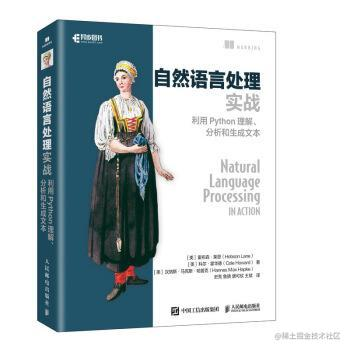[美] 霍布森·莱恩，科尔·霍华德，汉纳斯·马克斯·哈普克 著，史亮，鲁骁，唐可欣，王斌 译

• Python自然语言处理NLP入门到实战
• 兼具基础理论与编程实战，现代自然语言处理领域从业者的实用指南
• 提供源代码，小米AI实验室NLP团队献译